# RD Sharma Solutions for Class 6 Maths Chapter 18: Basic Geometrical Tools Exercise 18.1

Exercise 18.1 of Chapter 18 provides the students with knowledge about Geometrical Tools like ruler, dividers, compasses, protractor and set squares. It consists of questions based on the steps followed in the construction of shapes using set squares. The solutions are prepared in a simple language based on the understanding ability of the students. To perform well in the exam and score good marks students can use RD Sharma Solutions Class 6 Maths Chapter 18 Basic Geometrical Tools Exercise 18.1 PDF from the links which are provided below.

## RD Sharma Solutions for Class 6 Maths Chapter 18: Basic Geometrical Tools Exercise 18.1 Download PDF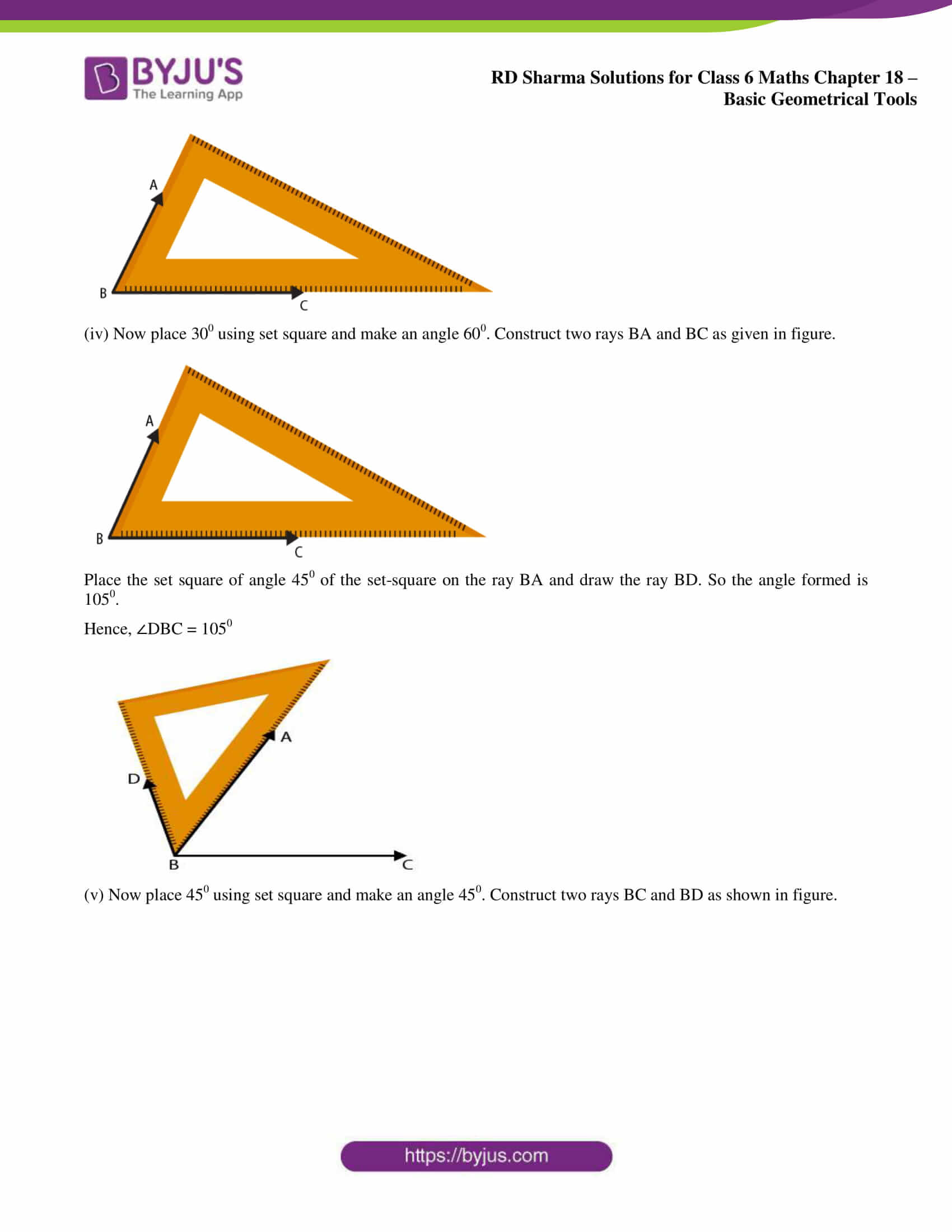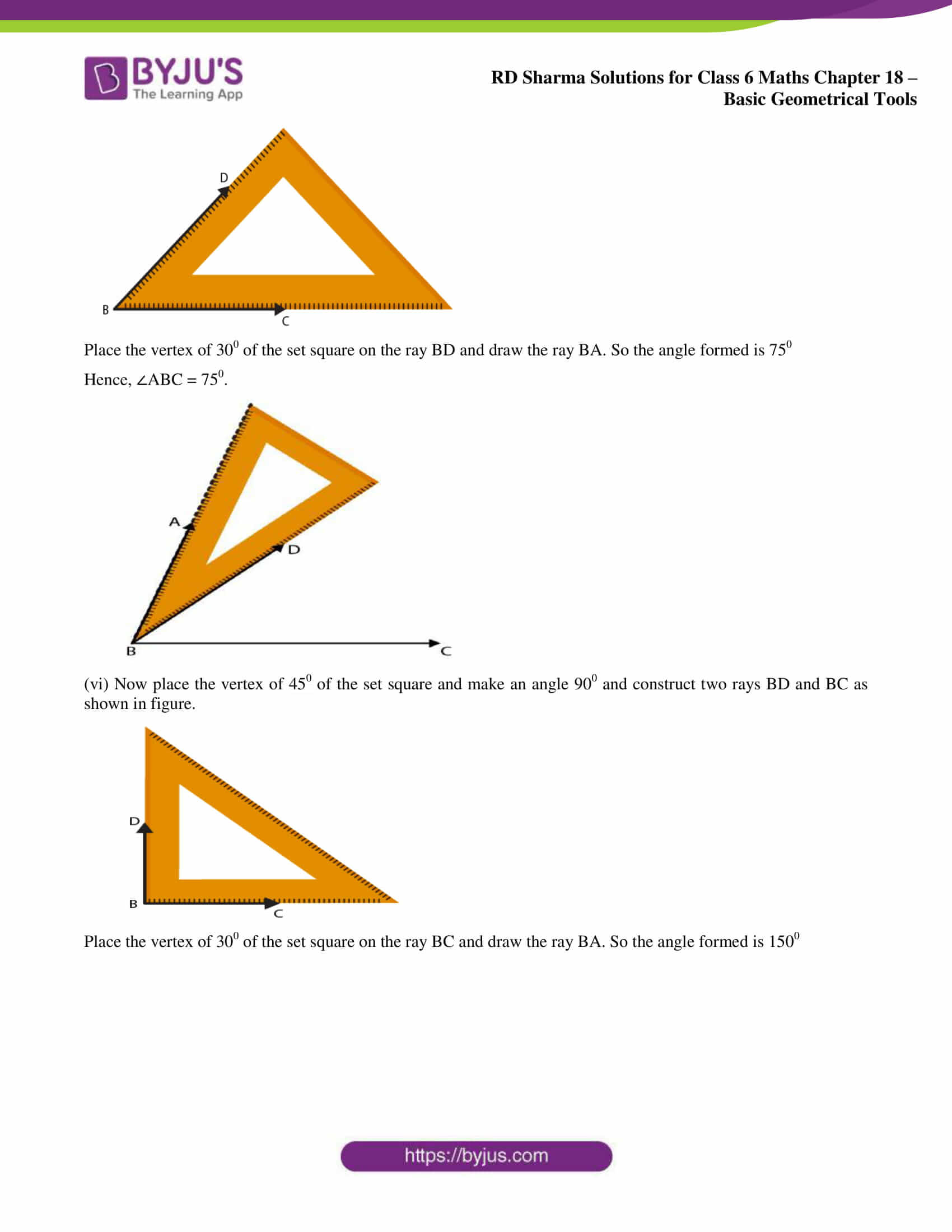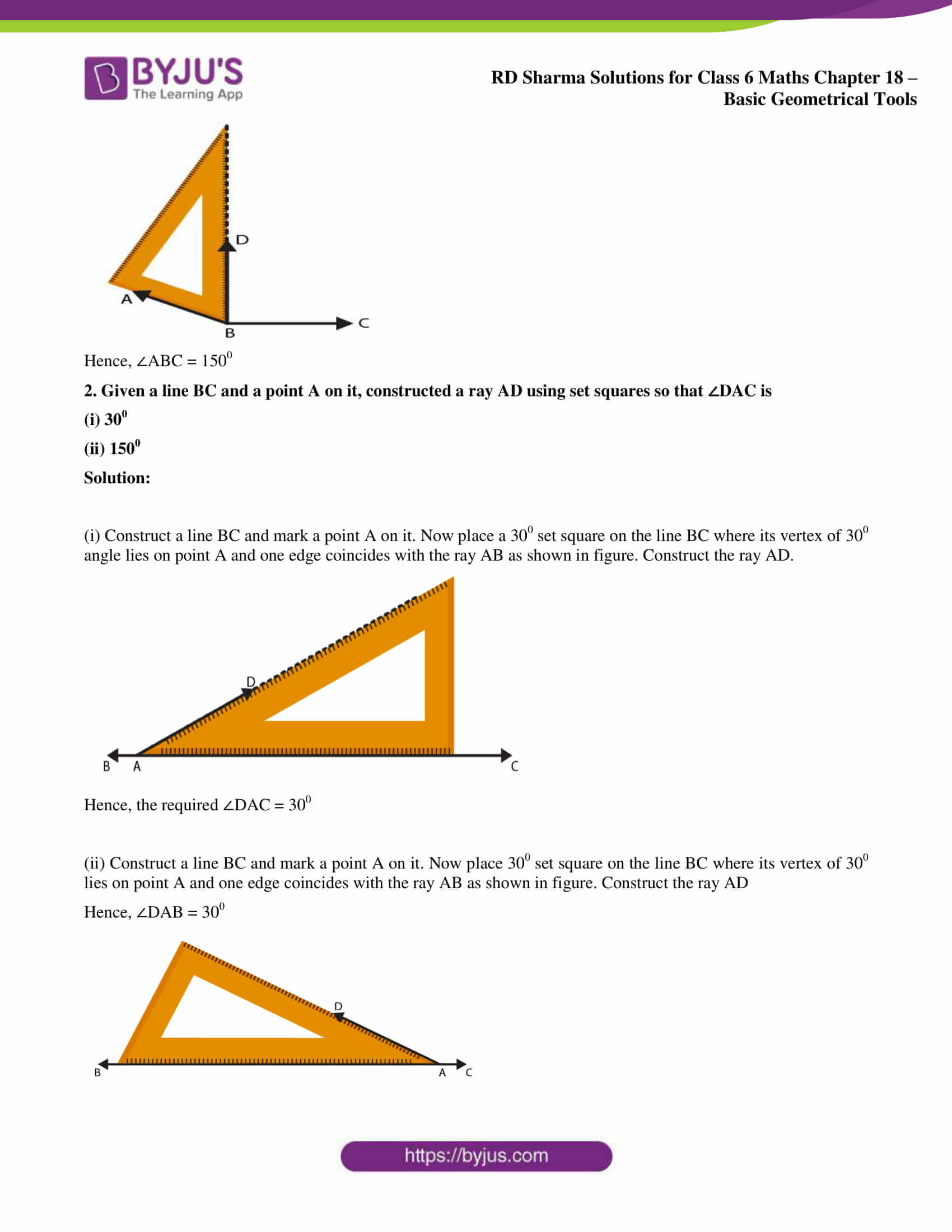### Exercise 18.1 page: 18.3

1. Construct the following angles using set-squares:

(i) 450

(ii) 900

(iii) 600

(iv) 1050

(v) 750

(vi) 1500

Solution:

(i) Now place 450 using set square. Construct two rays AB and AC along the edges from the vertex A of 450 angle of the set square. So the angle formed is 450

Hence, ∠BAC = 450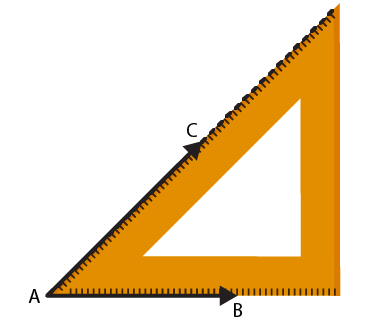(ii) Now place 900 using set square. Construct two rays BC and BA along the edges from the vertex B of 900 angle of the set square. So the angle formed is 900

Hence, ∠ABC = 900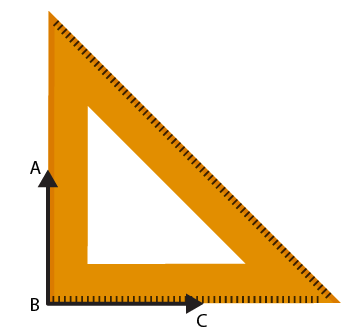g

(iii) Now place 600 using set square. Construct two rays BC and BA along the edges from the vertex B of 600 angle of the set square. So the angle formed is 600

Hence, ∠ABC = 600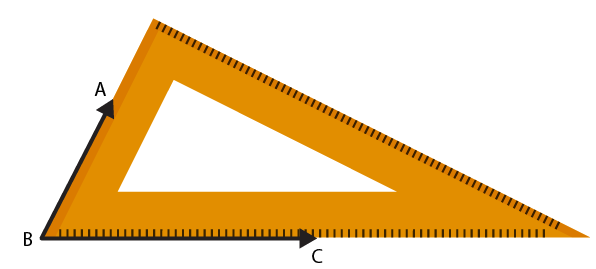(iv) Now place 300 using set square and make an angle 600. Construct two rays BA and BC as given in figure.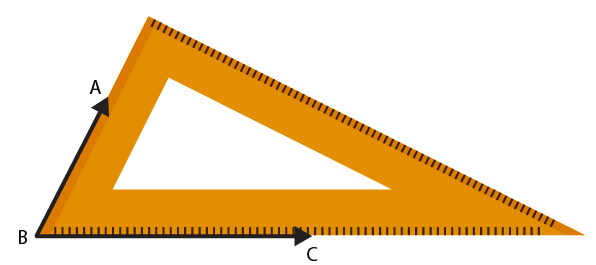Place the set square of angle 450 of the set-square on the ray BA and draw the ray BD. So the angle formed is 1050.

Hence, ∠DBC = 1050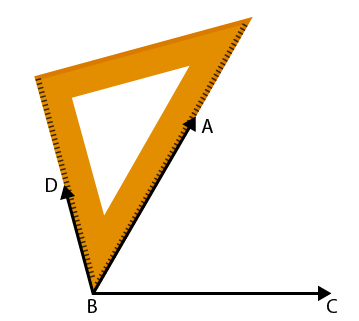(v) Now place 450 using set square and make an angle 450. Construct two rays BC and BD as shown in figure.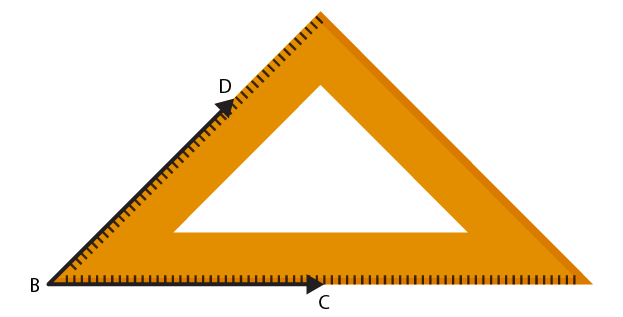Place the vertex of 300 of the set square on the ray BD and draw the ray BA. So the angle formed is 750

Hence, ∠ABC = 750.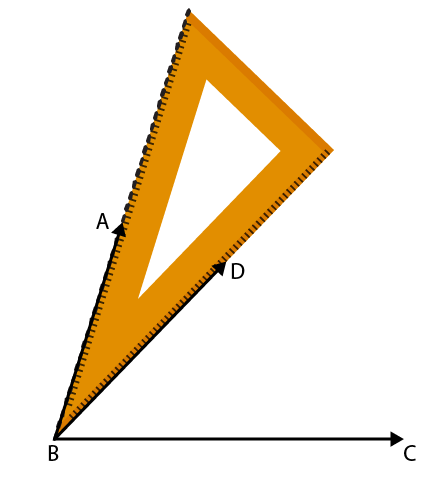(vi) Now place the vertex of 450 of the set square and make an angle 900 and construct two rays BD and BC as shown in figure.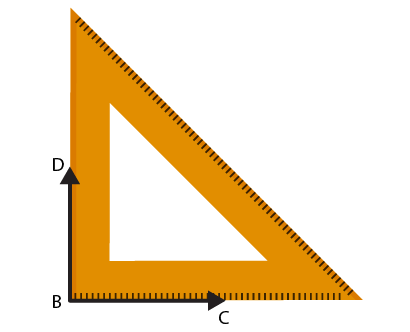Place the vertex of 300 of the set square on the ray BC and draw the ray BA. So the angle formed is 1500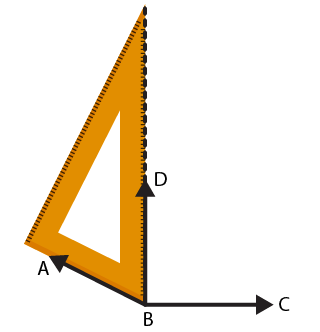Hence, ∠ABC = 1500

2. Given a line BC and a point A on it, constructed a ray AD using set squares so that ∠DAC is

(i) 300

(ii) 1500

Solution:

(i) Construct a line BC and mark a point A on it. Now place a 300 set square on the line BC where its vertex of 300 angle lies on point A and one edge coincides with the ray AB as shown in figure. Construct the ray AD.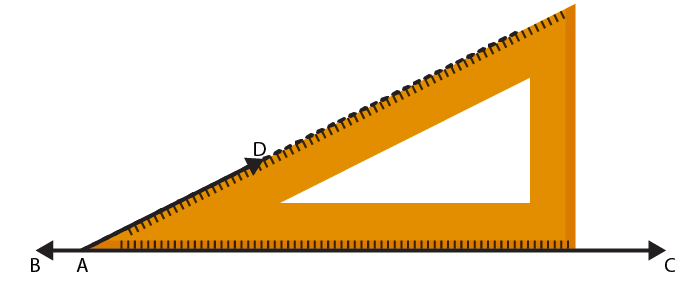Hence, the required ∠DAC = 300

(ii) Construct a line BC and mark a point A on it. Now place 300 set square on the line BC where its vertex of 300 lies on point A and one edge coincides with the ray AB as shown in figure. Construct the ray AD

Hence, ∠DAB = 300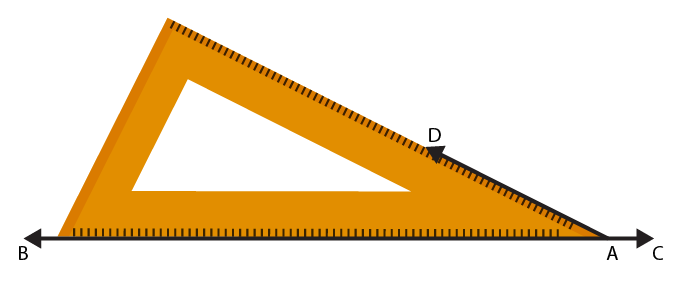We know that the angle on one side of the straight line will always add to 1800

So we get ∠DAB + ∠DAC = 1800

Hence, ∠DAC = 1500